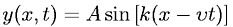Equations > Physics > Oscillations and Waves > Harmonic wave function

### Harmonic wave functionLatex Code:

MathML Code:

 $y\left(x,t\right)=A\mathrm{sin}\left[k\left(x-\upsilon t\right)\right]$

MathType 5.0: